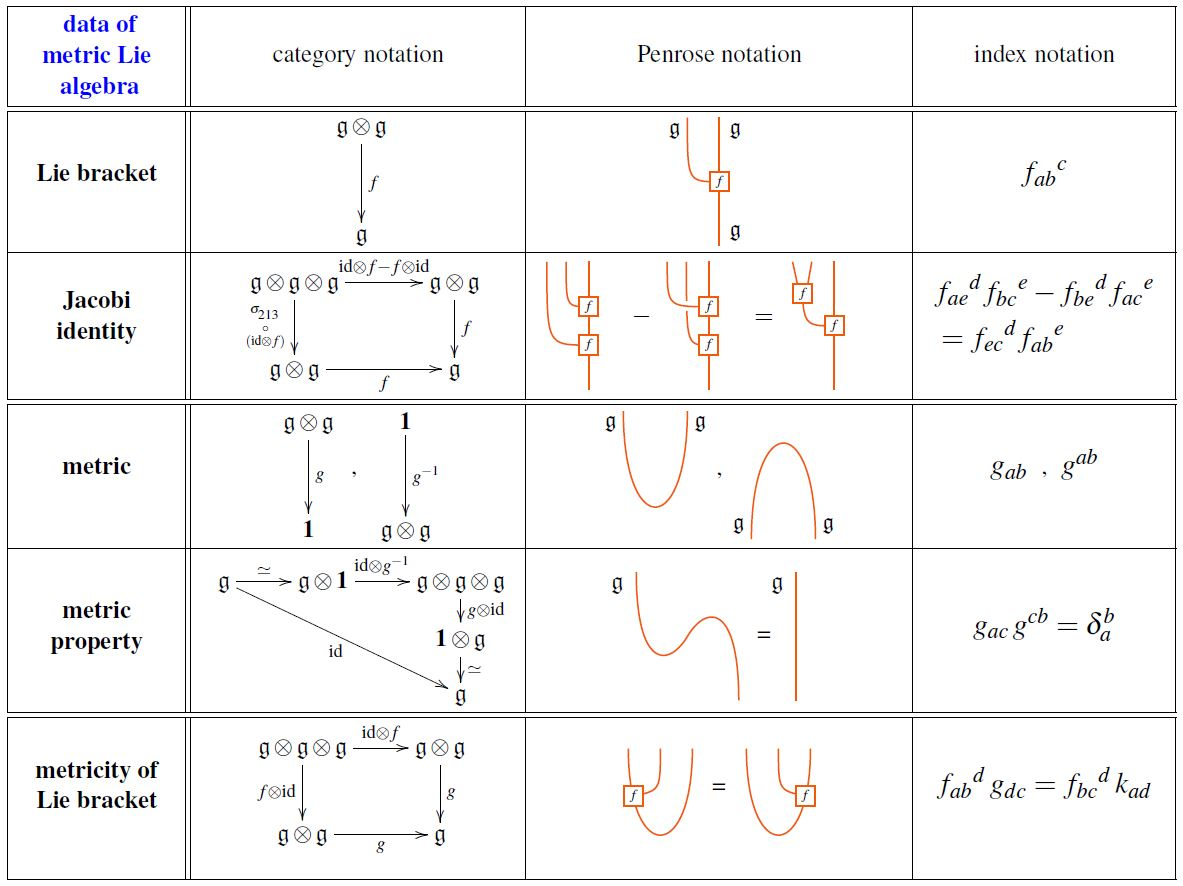Contents

# Contents

## Defintion

A metric Lie algebra or quadratic Lie algebra over some ground field $\mathbb{F}$ is

1. Lie algebra $\mathfrak{g}$

2. a bilinear form $\mathfrak{g} \otimes \mathfrak{g} \overset{g}{\longrightarrow} \mathbb{F}$

such that $g$

1. is symmetric and invariant under the adjoint action, hence is an invariant polynomial on $\mathfrak{g}$;

2. is non-degenerate as a bilinear form, in that its tensor product-adjunct $\mathfrak{g} \to \mathfrak{g}^\ast$ is a linear isomorphism.

The following table shows the data in a metric Lie representation equivalently

1. in category theory-notation;

2. in index notation:graphics from Sati-Schreiber 19c

## Applications

### Lie algebra weight systems on chord diagrams

“Most” weight systems on chord diagrams come from metric Lie representations over metric Lie algebras: these are the Lie algebra weight systems.

### General

On the Faulkner construction:

### Relation to M2-brane 3-Lie algebras

The full generalized axioms on the M2-brane 3-algebra and first insights into their relation to Lie algebra representations of metric Lie algebras is due to

The full identification of M2-brane 3-algebras with dualizable Lie algebra representations over metric Lie algebras is due to

reviewed in

• José Figueroa-O'Farrill, slide 145 onwards in: Triple systems and Lie superalgebras in M2-branes, ADE and Lie superalgebras, talk at IPMU 2009 (pdf, pdf)

further explored in

and putting to use the Faulkner construction that was previously introduced in (Faulkner 73)